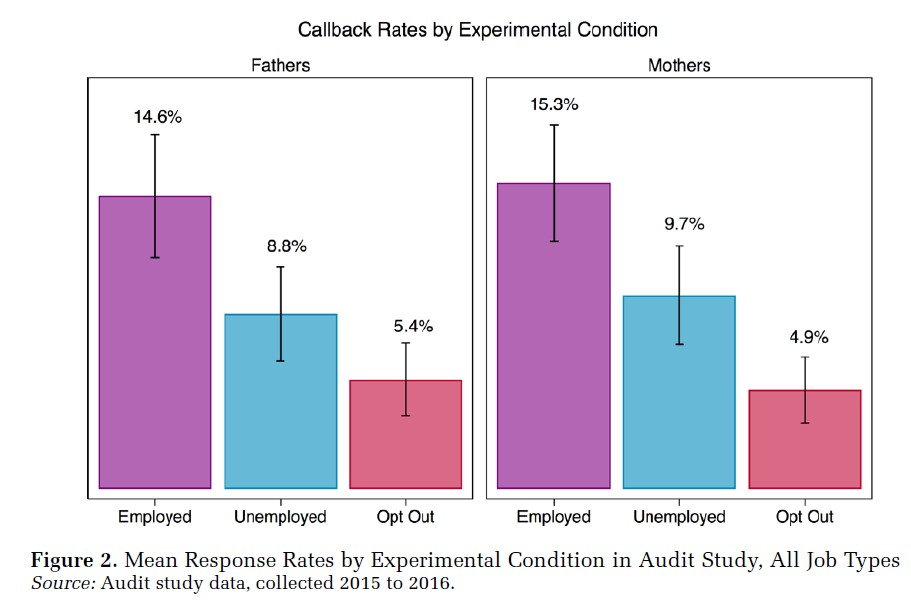Fall 2019

## Goals of the course

By the end of the course, you should know how to:

1. Import and manage data in R
2. Perform basic descriptive analysis
3. Create simple univariate and bivariate visualizations
4. Estimate and interpret basic regression models
5. Quantify the uncertainty of your conclusions
6. Make informed judgments about (simple) competing models
7. Create reproducible reports using R Markdown

• base R vs. tidyverse
• for tidyverse code, see link in Sakai
• you will learn tidyverse via DataCamp (custom track)
• for visualization basics, see http://socviz.co

## Things to be aware of

• R and RStudio
• R Markdown
• Slack
• DataCamp

## A typical week

Tuesday: lecture (possible quiz)

Wednesday: reread chapter; go through chapter code

Thursday: ask questions in lab, practice coding

Friday-Sunday: complete exercises, submit via Sakai

## Causes and potential outcomes

• Does more education cause higher wages?
• Does participating in a job training program cause a higher probability of employment?
• Do boycotts cause a drop in a company’s share price?
• These are tough questions!

## Threats to causal inference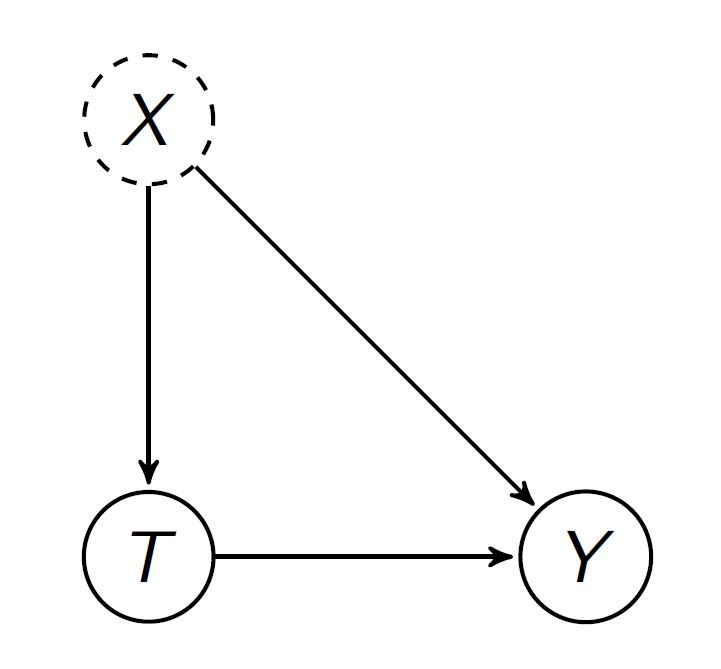## Key term: Identification

How do we identify the effect of a treatment (cause) on an outcome?

## Experiments and the “independent variable”

• What is an experiment?
• How do experiments solve the problem we just talked about?

## Example: Audit study

• Correll et al. sent fake resumes and cover letters to 300+ employers
• Treatment categories
• Mother (PTA officer; relocating “with family”)
• Childless (alumni officer; relocating)

## Results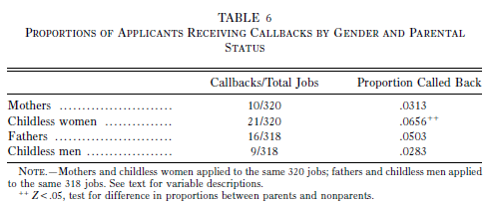They find a negative effect of motherhood on the probability of a call back

## Results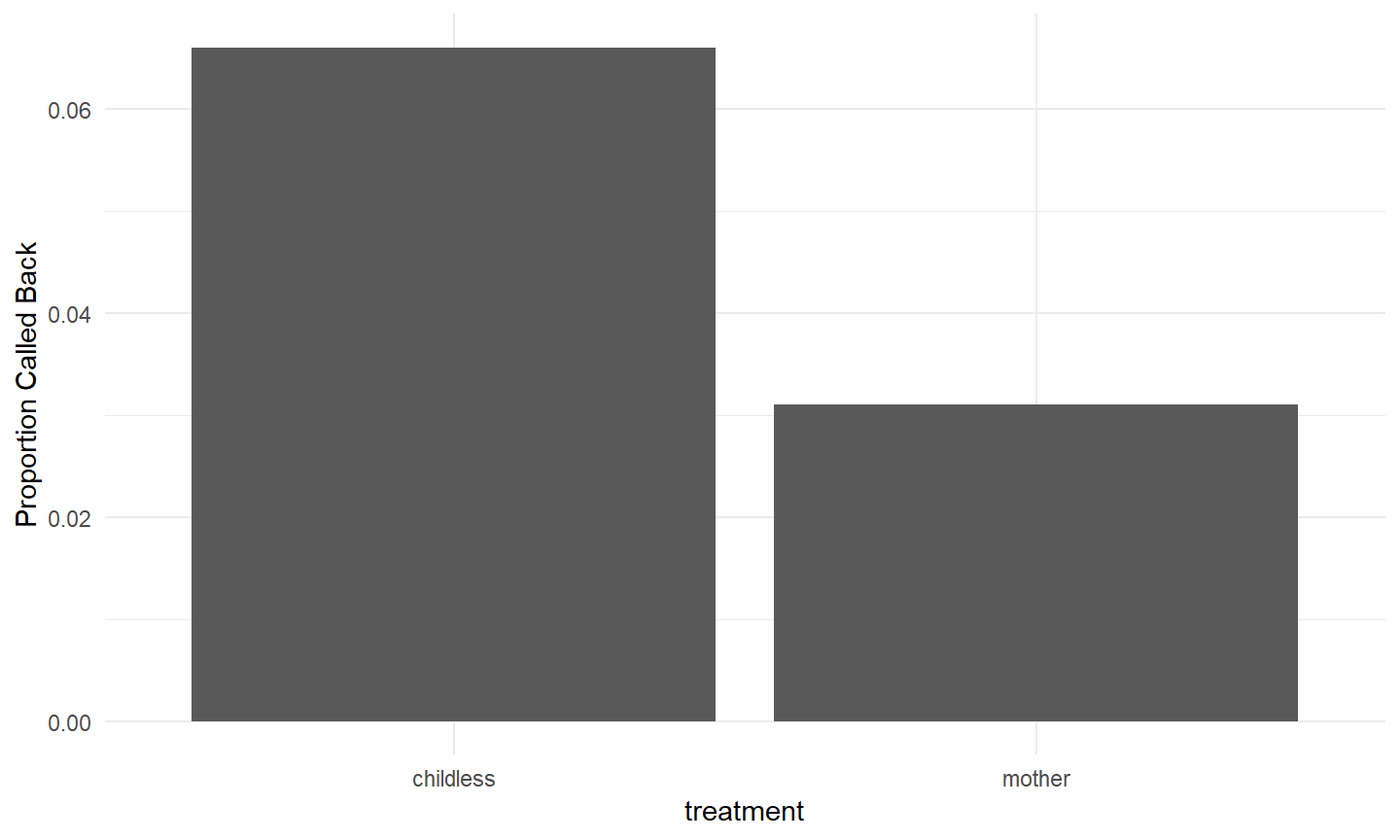## Experiments are great!

Assuming successful randomization to treatment and control, you know it’s the treatment that’s causing the effect.

## Experiments can’t do everything

• ethics
• external validity
• often non-representative
• some treatments are hard or impossible to assign randomly
• motherhood
• divorce
• boycotts

## Some notation

$$T$$ a binary treatment variable

$$Y$$ the value of the outcome we observe

$$Y^0$$ the value the outcome would take if $$T=1$$

$$Y^1$$ the value the outcome would take if $$T=0$$

Let’s think about the last two a bit more carefully…

## The world before the experiment

Subject $$Y^0$$ $$Y^1$$ $$T$$ $$Y$$
Andrew 2 3
Barb 3 4
Catherine 3 4
David 2 3

What do these numbers mean?

## The world after the experiment

Subject $$Y^0$$ $$Y^1$$ $$T$$ $$Y$$
Andrew 3 1 3
Barb 3 0 3
Catherine 4 1 4
David 2 0 2

$Y = TY^1+(1-T)Y^0$

$$Y = Y^1$$ for $$T = 1$$

$$Y = Y^0$$ for $$T = 0$$

We can’t know $$Y^1$$ for those who are $$T=0$$

We can’t know $$Y^0$$ for those who are $$T=1$$

## Potential outcomes and counterfactuals

$$Y^0$$ and $$Y^1$$ are potential outcomes

In the real world, $$T$$ is either 1 or 0 for each case.

We see $$Y^1$$ or $$Y^0$$, but never both.

When $$T=0$$, $$Y^1$$ is counterfactual

When $$T=1$$, $$Y^0$$ is counterfactual

## What do we want to know?

We really care about the difference between $$Y^0$$ and $$Y^1$$. (Why?)

Let $$\delta_i = y^1_i - y^0_1$$

$$E[\delta]=E[Y^1-Y^0]$$

$$E[\delta]=E[Y^1]-E[Y^0]$$

## Assume an experiment: What is $$E[\delta]$$?

Subject $$Y^0$$ $$Y^1$$ $$T$$ $$Y$$
Andrew 2 3
Barb 3 4
Catherine 3 4
David 2 3

## Assume an experiment: What is $$E[\delta]$$?

Subject $$Y^0$$ $$Y^1$$ $$T$$ $$Y$$
Andrew 3 1 3
Barb 3 0 3
Catherine 4 1 4
David 2 0 2

## Why can we do this with experiments?

$$T \bot Y^0$$

$$T \bot Y^1$$

$$E[Y^0 | T = 0] = E[Y^0 | T = 1 ]$$

$$E[Y^1 | T = 0] = E[Y^1 | T = 1 ]$$

## Or in other words…

In a properly executed experiment, there is no association between the potential outcome variables and treatment assignment.

$$E[Y^0 | T = 0] \simeq E[Y^0]$$

$$E[Y^1 | T = 1] \simeq E[Y^1]$$

So…

$$E[\delta] = E[Y|T=1]-E[Y|T=0]$$

The difference between the treatment average and the control average

## What is this treatment effect?

$$E[\delta]$$ is the expected value (mean) of the difference between each unit’s value of $$Y^1$$ and $$Y^0$$. It is the average treatment effect (ATE). In a sample, this is the sample average treatment effect (SATE).

Even though the individual differences are unobservable (because either $$Y^0$$ or $$Y^1$$ will be counterfactual for each unit), we can estimate the mean difference via experiment.

$\text{SATE} = \frac{1}{n}\sum_{i=1}^{n}(y^1_i - y^0_i)$

## Randomization

• Experiments identify the SATE because cases are randomly assigned to the treatment and control group and are, therefore, identical on average, on all pre-treatment characteristics.

• Experiments are sometimes called randomized controlled trials (or RCTs)

## Internal and external validity

• Internal validity: the extent to which causal assumptions are satisfied in the study

• External validity: the extent to which the conclusions can be generalized beyond a particular study.

## Example: trolley problem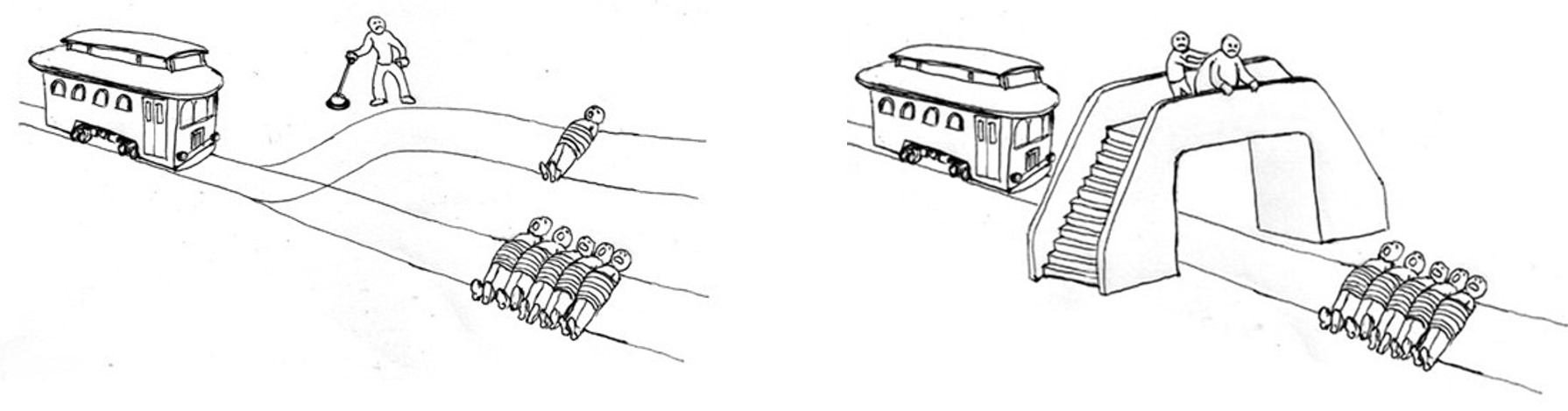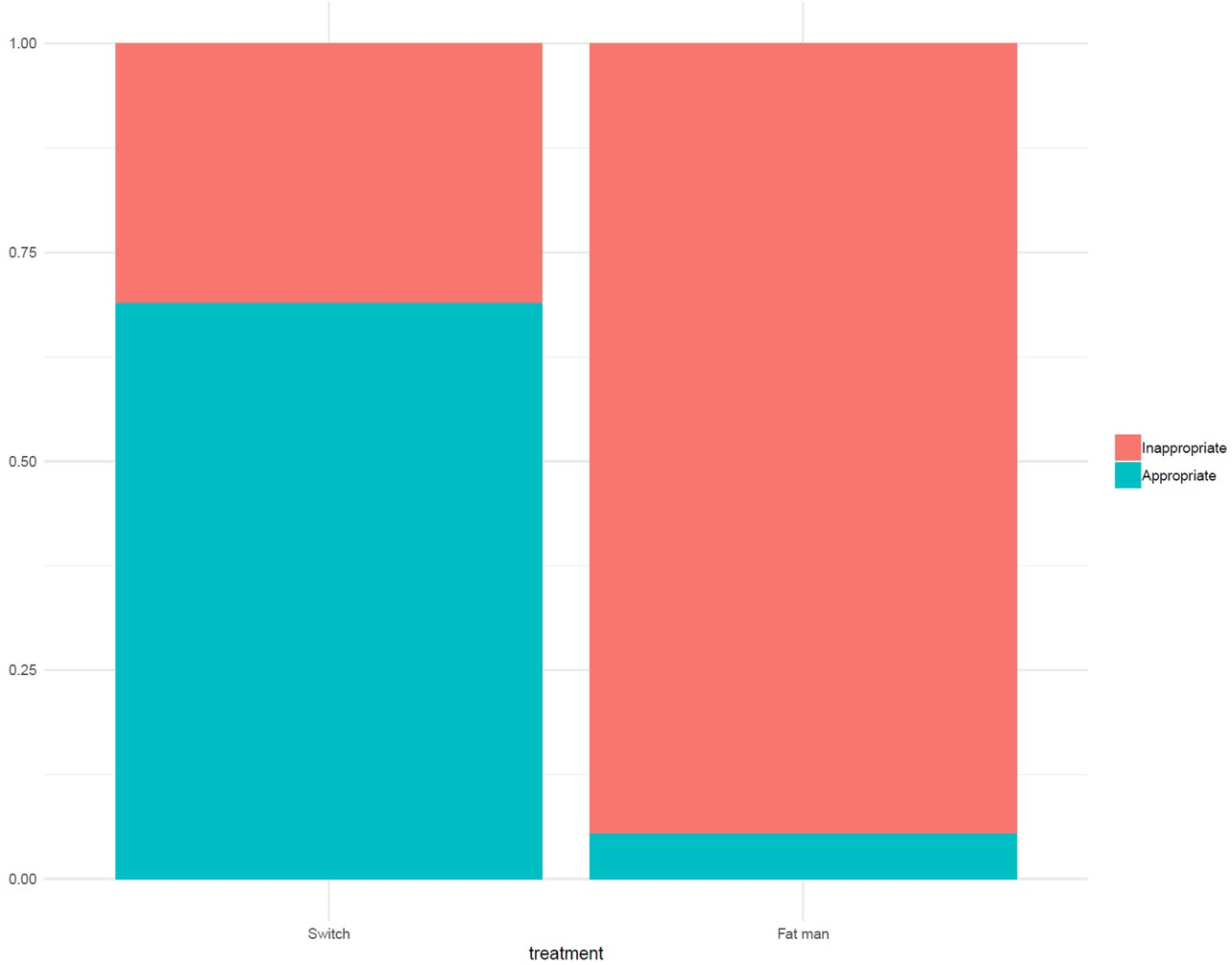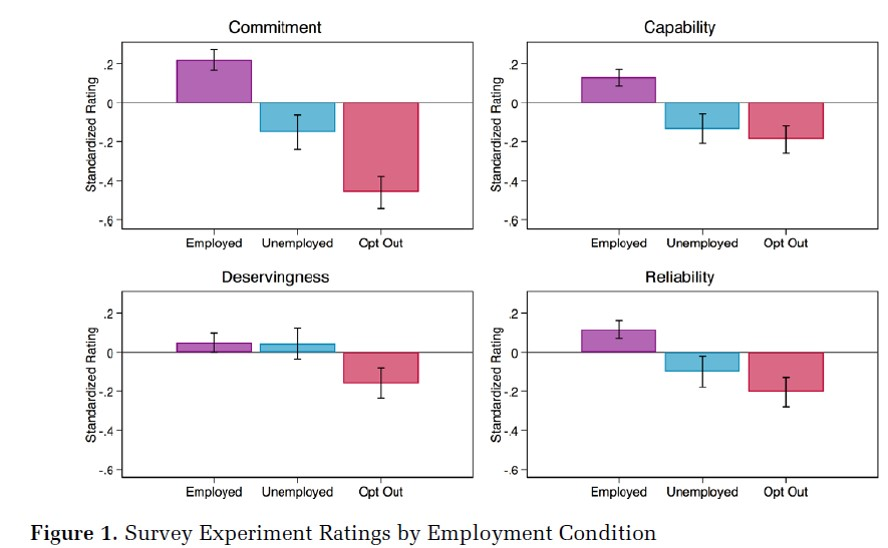Weisshaar, K. (2018). “From Opt Out to Blocked Out: The Challenges for Labor Market Re-entry after Family-Related Employment Lapses.” American Sociological Review, 83(1), 34–60.